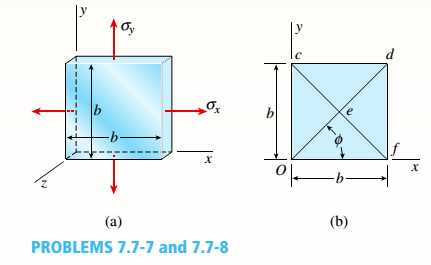# A thin square plate in biaxial stress is subjected to stresses ?? and ??., as shown in part a of the figure. The width of the plate is h = 12.0 in. Measurements show that the normal strains in the x and v directions are s = 427 × 10-6 and s = 113 × l0-6, respectively. With reference to part b of the figure. which shows a Iwo-dimensional view of the plate. determine the following quantities. (a) The increase .d in the length of diagonal Oil. (b) The change . in the angle between diagonal Oti and the x axis, (c) The shear strain y associated with diagonals Oil and cf(that is. find the decrease in angle ced).### Mechanics of Materials (MindTap Co...

9th Edition
Barry J. Goodno + 1 other
Publisher: Cengage Learning
ISBN: 9781337093347
Chapter 7, Problem 7.7.7P
Textbook Problem
27 views

## A thin square plate in biaxial stress is subjected to stresses ?? and ??., as shown in part a of the figure. The width of the plate is h = 12.0 in. Measurements show that the normal strains in the x and v directions are s = 427 × 10-6 and s = 113 × l0-6, respectively. With reference to part b of the figure. which shows a Iwo-dimensional view of the plate. determine the following quantities. (a) The increase .d in the length of diagonal Oil. (b) The change . in the angle between diagonal Oti and the x axis, (c) The shear strain y associated with diagonals Oil and cf(that is. find the decrease in angle ced).(a)

To determine

The increase in the length of the diagonal.

### Explanation of Solution

Given information:

The width of the square plate is 12.0 in , the strain along x-direction is 427 × 10 6 , the strain along y-direction is 113 × 10 6 and the angle of inclination is 45 ° .

Explanation:

Write the expression for the length of the diagonal.

L d = b 2 ...... (I)

Write the expression for strain along x 1 -direction.

ε x 1 = ε x + ε y 2 + ε x ε y 2 cos ( 2 θ ) + γ x y 2 sin ( 2 θ ) ...... (II)

Write the expression for change in diameter.

Δ d = ε x 1 L d ...... (III)

Calculation:

Substitute, 12 in for b in Equation (I).

L d = 12 in 2 = 12 in × 1.41421 = 16.97 in

Substitute, 427 × 10 6 for ε x , 113 × 10 6 for ε y

0 for γ x y and 45 ° for θ in Equation (II)

(b)

To determine

The change in the angle between diagonal Od and the x-axis.

(c)

To determine

The shear strain associated with the diagonals.

### Still sussing out bartleby?

Check out a sample textbook solution.

See a sample solution

#### The Solution to Your Study Problems

Bartleby provides explanations to thousands of textbook problems written by our experts, many with advanced degrees!

Get Started

Find more solutions based on key concepts
What is physical independence?

Database Systems: Design, Implementation, & Management

Solve Problem 20.17 using Excel.

Engineering Fundamentals: An Introduction to Engineering (MindTap Course List)

Determine the tension in each of the three ropes supporting the 800-lb crate.

International Edition---engineering Mechanics: Statics, 4th Edition

List the sections of a system design specification, and describe the contents.

Systems Analysis and Design (Shelly Cashman Series) (MindTap Course List)

What are some other reasons why you might need to know the operating system version on your computer or mobile ...

Enhanced Discovering Computers 2017 (Shelly Cashman Series) (MindTap Course List)

What are the best ways to remove slag between filler weld passes?

Welding: Principles and Applications (MindTap Course List)

If your motherboard supports ECC DDR3 memory, can you substitute non-ECC DDR3 memory?

A+ Guide to Hardware (Standalone Book) (MindTap Course List)